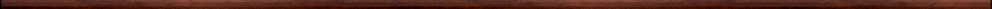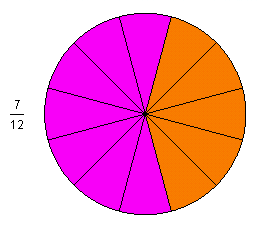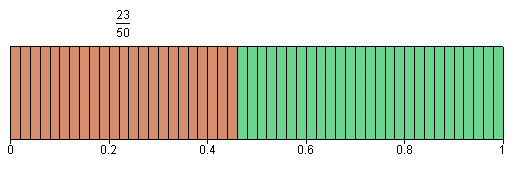Maple worksheets on illustrating fractions and operations on fractionsIntroductory, general and miscellaneous worksheets:

They are all compatible with Classic Worksheet Maple 10.Pictorial representation of fractions - draw_fraction.mws

• A procedure for drawing pictures to illustrate fractions: DrawFraction
• Examples

• A procedure for drawing pictures to illustrate the addition of fractions: AddFractions
• Examples

Pictorial comparison of fractions and subtraction of fractions - comp_fraction.mws

• A procedure for drawing pictures to illustrate the comparison of fractions: CompareFractions
• Examples

Pictorial representation of multiplication of fractions - mult_fraction.mws

• A procedure for drawing pictures to illustrate the multiplication of fractions: MultFractions
• Examples

Procedures for fractions - fractions.zipTop of page

Main index